Next: Bibliography Up: MCMC Previous: Global class proportion parameters,

### Continuous weights parameters,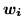This is sampled from using Metropolis-Hastings. We loop through all voxels sampling from the continuous weights vector a voxel at a time. Recall that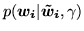is the deterministic logistic transform in equation 12 at voxel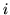. The logistic transform can only take us from a vector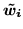to a vector. Hence, in practice we propose jumps onand then calculate the vectorthis gives us. Then, for model 1 we need to recalculate: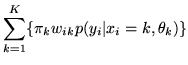(32)

or for models 2 and 3: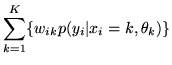(33)

Plus for models 2 and 3 we also need to update the parts of the MRF prior that change when we change. These are: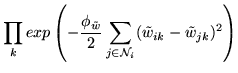(34)

whereis the set of voxels in the spatial neighbourhood of voxel.Next: Bibliography Up: MCMC Previous: Global class proportion parameters,